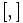# Lie ring whose bracket is the double of a Lie bracket giving nilpotency class two

This article defines a Lie ring property: a property that can be evaluated to true/false for any Lie ring.
View a complete list of properties of Lie rings
VIEW RELATED: Lie ring property implications | Lie ring property non-implications |Lie ring metaproperty satisfactions | Lie ring metaproperty dissatisfactions | Lie ring property satisfactions | Lie ring property dissatisfactions

## Definition

Suppose$L$ is a Lie ring with Lie bracket$[ , ]$. We say that$L$ is the Lie ring whose bracket is the double of a Lie bracket giving nilpotency class two if there exists a Lie bracket$\{ , \}$ with the same set$L$ and the same additive group structure, such that both these conditions are satisfied:

1.$2 \{ x, y \} = [x,y] \ \forall \ x,y \in L$
2. With the Lie bracket$\{ , \}$,$L$ is a Lie ring of nilpotency class two (i.e., its nilpotency class is at most two).

Note that this implies that$L$, with the original Lie bracket, is also of nilpotency class at most two.

## Relation with other properties

### Stronger properties

Property Meaning Proof of implication Proof of strictness (reverse implication failure) Intermediate notions
abelian Lie ring Lie bracket is trivial (take the half to be the trivial Lie bracket too) CS-Baer Lie ring, LCS-Baer Lie ring|FULL LIST, MORE INFO
Baer Lie ring Lie ring of class at most two that is also uniquely 2-divisible, i.e., every element has a unique half define$\{ x,y \} = \frac{1}{2}[x,y]$ CS-Baer Lie ring, LCS-Baer Lie ring|FULL LIST, MORE INFO
LCS-Baer Lie ring Lie ring of class at most two whose derived subring is uniquely 2-divisible CS-Baer Lie ring|FULL LIST, MORE INFO